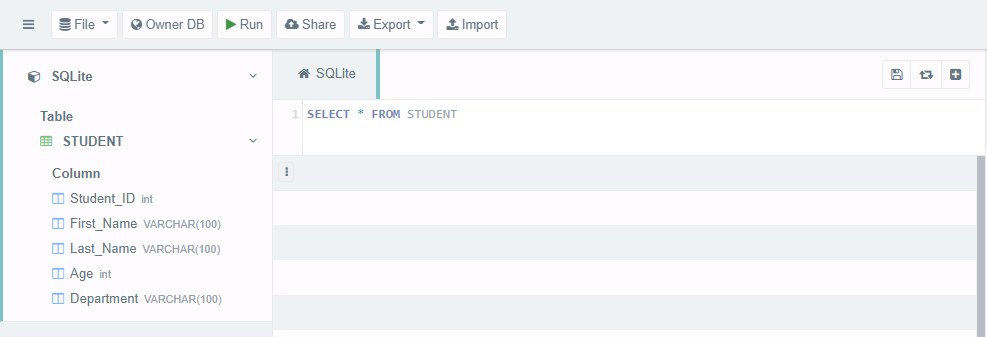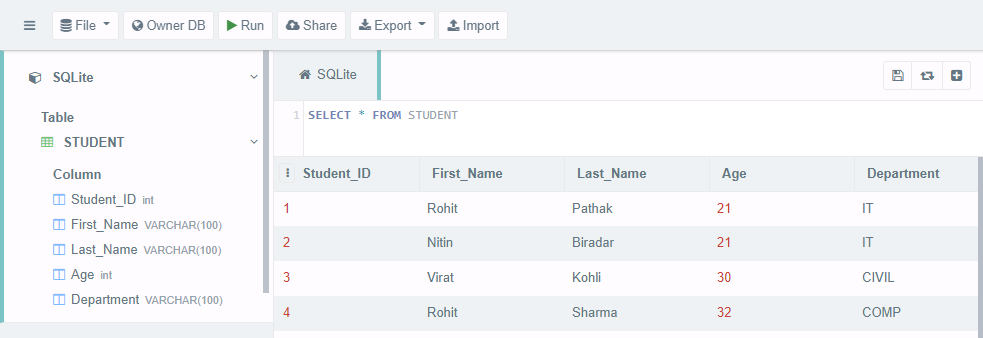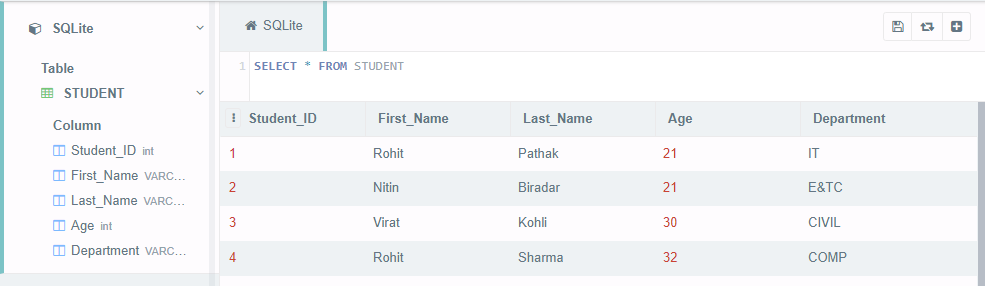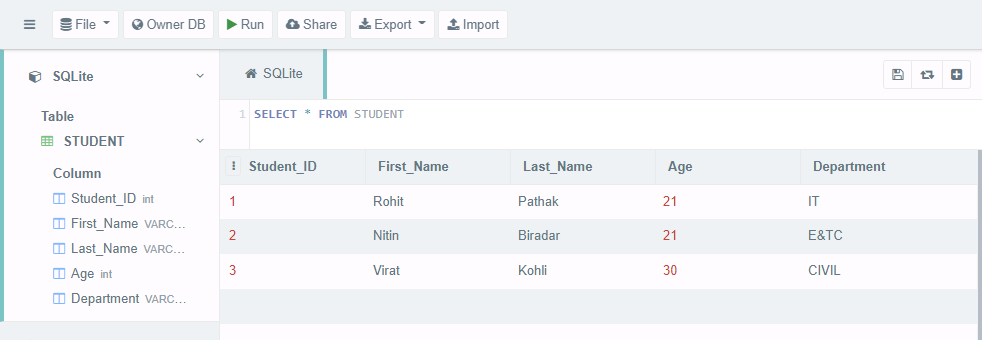# Python SQLite – WHERE Clause

Where clause is used in order to make our search results more specific, using the where clause in SQL/SQLite we can go ahead and specify specific conditions that have to be met when retrieving data from the database.

If we want to retrieve, update or delete a particular set of data we can use the where clause. If we don’t have condition matching values in your database tables we probably didn’t get anything returned.

WHERE Clause in SQL:

Syntax:

SELECT column_1, column_2,…,column_N

FROM table_name

WHERE [search_condition]

Here, in this [search_condition] you can use comparison or logical operators to specify conditions.

For example:  = , > , < , != , LIKE, NOT, etc.

### WHERE Clause in SQLite using Python:

In Python SQLite Cursor object/class which contains all the methods to execute SQL queries to perform operations, etc. The Cursor is the method of connection class that returns a cursor object.

Therefore, to perform SQLite commands in python we need 3 basic things to be done −

• Establish a connection with the database using the connect() method.
• Create a cursor object by using the cursor() method.
• Now SQLite queries/statements can be executed using the execute() method of the Cursor class.

We’ll create a database to manage data about students. We’ll be storing information about each student, and then we’ll also create a way to retrieve, update and delete data of students using the where clause.

Let’s Creates Database (geekforgeeks_student.db) and a Table (STUDENT).

## Python3

 `# import the sqlite3 module ` `import` `sqlite3 ` ` `  `# Define connection and cursor ` `connection ``=` `sqlite3.connect(``'geekforgeeks_student.db'``) ` `cursor ``=` `connection.cursor() ` ` `  `# create table ` `cursor.execute(``"DROP TABLE IF EXISTS STUDENT"``) ` `createTable ``=` `'''CREATE TABLE STUDENT( ` `   ``Student_ID int, First_Name VARCHAR(100), ` `   ``Last_Name VARCHAR(100), Age int, ` `   ``Department VARCHAR(100) ` `)'''` `cursor.execute(createTable) ` ` `  `# check the database creation data ` `if` `cursor: ` `    ``print``(``"Database Created Successfully !"``) ` `else``: ` `    ``print``(``"Database Creation Failed !"``) ` ` `  `# Commit the changes in database and Close the connection ` `connection.commit() ` `connection.close()`

Output:

`Database Created Successfully!`

The above code will create “geekforgeeks_student.db” file locally.To view the content of the “geekforgeeks_student.db” we can use this and load our .db file as shown below –In the above image, we can see the table and table schema has been created in the database.

Now we will insert data into STUDENT table.

## Python3

 `# import the sqlite3 module ` `import` `sqlite3 ` ` `  `# Define connection and cursor ` `connection ``=` `sqlite3.connect(``'geekforgeeks_student.db'``) ` `cursor ``=` `connection.cursor() ` ` `  `# Insert data into the table ` `cursor.execute(``"INSERT INTO STUDENT VALUES (1,'Rohit', 'Pathak', 21, 'IT')"``) ` `cursor.execute(``"INSERT INTO STUDENT VALUES (2,'Nitin', 'Biradar', 21, 'IT')"``) ` `cursor.execute(``"INSERT INTO STUDENT VALUES (3,'Virat', 'Kohli', 30, 'CIVIL')"``) ` `cursor.execute(``"INSERT INTO STUDENT VALUES (4,'Rohit', 'Sharma', 32, 'COMP')"``) ` ` `  `# printing the cursor data ` `if` `cursor: ` `    ``print``(``"Data Inserted !"``) ` `else``: ` `    ``print``(``"Data Insertion Failed !"``) ` ` `  `# Commit the changes in database and Close the connection ` `connection.commit() ` `connection.close() `

Output:

`Database Inserted!`

The above code will insert the data into the STUDENT tableThe following codes show the use of Where Clause

Example 1: To retrieve the data of the students whose Department is IT

## Python3

 `import` `sqlite3 ` ` `  `connection ``=` `sqlite3.connect(``'geekforgeeks_student.db'``) ` `cursor ``=` `connection.cursor() ` ` `  `# WHERE CLAUSE TO RETRIEVE DATA ` `cursor.execute(``"SELECT * FROM STUDENT WHERE Department = 'IT'"``) ` ` `  `# printing the cursor data ` `print``(cursor.fetchall()) ` ` `  `connection.commit() ` `connection.close() `

Output:

[(1, ‘Rohit’, ‘Pathak’, 21, ‘IT’), (2, ‘Nitin’, ‘Biradar’, 21, ‘IT’)]

We have 2 records in STUDENT table whose Department is IT.

Example 2: To retrieve the data of the students whose First Name starts with ‘R’. We can also use Wildcard characters with where clause as shown below

## Python3

 `import` `sqlite3 ` ` `  `connection ``=` `sqlite3.connect(``'geekforgeeks_student.db'``) ` `cursor ``=` `connection.cursor() ` ` `  `# WHERE CLAUSE TO RETRIEVE DATA ` `cursor.execute(``"SELECT * from STUDENT WHERE First_name Like'R%'"``) ` ` `  `# printing the cursor data ` `print``(cursor.fetchall()) ` ` `  `connection.commit() ` `connection.close() `

Output:

[(1, ‘Rohit’, ‘Pathak’, 21, ‘IT’), (4, ‘Rohit’, ‘Sharma’, 32, ‘COMP’)]

We have 2 records in the STUDENT table whose First Name starts with the letter ‘R’.

Example 3: To update the data of student whose Student ID is 4

## Python3

 `import` `sqlite3 ` ` `  `connection ``=` `sqlite3.connect(``'geekforgeeks_student.db'``) ` `cursor ``=` `connection.cursor() ` ` `  `# WHERE CLAUSE TO UPDATE DATA ` `cursor.execute(``"UPDATE STUDENT SET Department ='E&TC' WHERE Student_ID = 2"``) ` ` `  `# printing the cursor data ` `cursor.execute(``"SELECT * from STUDENT"``) ` `print``(cursor.fetchall()) ` ` `  `connection.commit() ` `connection.close() `

Output:

[(1, ‘Rohit’, ‘Pathak’, 21, ‘IT’), (2, ‘Nitin’, ‘Biradar’, 21, ‘E&TC’),

(3, ‘Virat’, ‘Kohli’, 30, ‘CIVIL’), (4, ‘Rohit’, ‘Sharma’, 32, ‘COMP’)]

Check the database contentDepartment is updated for Student ID 2.

Example 4: To Delete the data of student whose Age ID is 30

## Python3

 `import` `sqlite3 ` ` `  `connection ``=` `sqlite3.connect(``'geekforgeeks_student.db'``) ` `cursor ``=` `connection.cursor() ` ` `  `# WHERE CLAUSE TO DELETE DATA ` `cursor.execute(``"DELETE from STUDENT WHERE Age = 32"``) ` ` `  `#printing the cursor data ` `cursor.execute(``"SELECT * from STUDENT"``) ` `print``(cursor.fetchall()) ` ` `  `connection.commit() ` `connection.close()`

Output:

[(1, ‘Rohit’, ‘Pathak’, 21, ‘IT’), (2, ‘Nitin’, ‘Biradar’, 21, ‘E&TC’), (3, ‘Virat’, ‘Kohli’, 30, ‘CIVIL’)]

Check the database contentData of Student whose Age is 32 has been deleted.

Whether you're preparing for your first job interview or aiming to upskill in this ever-evolving tech landscape, GeeksforGeeks Courses are your key to success. We provide top-quality content at affordable prices, all geared towards accelerating your growth in a time-bound manner. Join the millions we've already empowered, and we're here to do the same for you. Don't miss out - check it out now!

Previous
Next